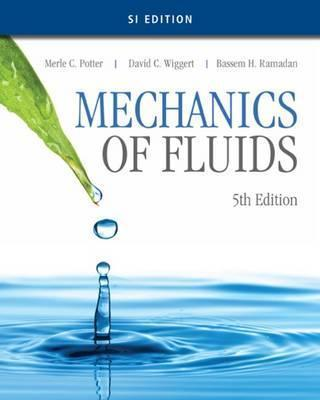# MECHANICS OF FLUIDS 5E SI POTTER 9781305637610

RM 108.00

TITLE : MECHANICS OF FLUIDS - POTTER

ISBN13 : 9781305637610

PUBLISHER : CENGAGE (2016)

EDITION : 5E SI UNIT PAPERBACK

PAGES : 793 PAGES

Gain an understanding of fluid mechanics and the ability to analyze this important phenomena encountered by practicing engineers with MECHANICS OF FLUIDS, 5E. The authors use proven learning tools to help you visualize many difficult-to-understand aspects of fluid mechanics. The mathematics used in derivations are readily accessible to you as an undergraduate engineering student.

1. BASIC CONSIDERATIONS.
Introduction. Dimensions, Units, and Physical Quantities. Continuum View of Gases and Liquids. Pressure and Temperature Scales. Fluid Properties. Conservation Laws. Thermodynamic Properties and Relationships. Summary. Problems.
2. FLUID STATICS.
Introduction. Pressure at a Point. Pressure Variation. Fluids at Rest. Linearly Accelerating Containers. Rotating Containers. Summary. Problems.
3. INTRODUCTION TO FLUIDS IN MOTION.
Introduction. Description of Fluid Motion. Classification of Fluid Flows. The Bernoulli Equation. Summary. Problems.
4. THE INTEGRAL FORMS OF THE FUNDAMENTAL LAWS.
Introduction. The Three Basic Laws. System-to-Control-Volume Transformation. Conservation of Mass. Energy Equation. Momentum Equation. Moment-of-Momentum Equation. Summary. Problems.
5. THE DIFFERENTIAL FORMS OF THE FUNDAMENTAL LAWS.
Introduction. Differential Continuity Equation. Differential Momentum Equation. Differential Energy Equation. Summary. Problems.
6. DIMENSIONAL ANALYSIS AND SIMILITUDE.
Introduction. Dimensional Analysis. Similitude. Normalized Differential Equations. Summary. Problems.
7. INTERNAL FLOWS.
Introduction. Entrance Flow and Developed Flow. Laminar Flow in a Pipe. Laminar Flow between Parallel Plates. Laminar Flow between Rotating Cylinders. Turbulent Flow in a Pipe. Uniform Turbulent Flow in Open Channels. Summary. Problems.
8. EXTERNAL FLOWS.
Introduction. Separation. Flow Around Immersed Bodies. Lift and Drag on Airfoils. Potential-Flow Theory. Boundary-Layer Theory. Summary. Problems.
9. COMPRESSIBLE FLOW.
Introduction. Speed of Sound and the Mach Number. Isentropic Nozzle Flow. Normal Shock Wave. Shock Waves in Converging-Diverging Nozzles. Vapor Flow through a Nozzle. Oblique Shock Wave. Isentropic Expansion Waves. Summary. Problems.
10. FLOW IN OPEN CHANNELS.
Introduction. Open-Channel Flows. Uniform Flow. Energy Concepts. Momentum Concepts. Nonuniform Gradually Varied Flow. Numerical Analysis of Water Surface Profiles. Summary. Problems.
11. FLOWS IN PIPING SYSTEMS.
Introduction. Losses in Piping Systems. Simple Pipe Systems. Analysis of Pipe Networks. Unsteady Flow in Pipelines. Summary. Problems.
12. TURBOMACHINERY.
Introduction. Turbopumps. Dimensional Analysis and Similitude for Turbomachinery. Use of Turbopumps in Piping Systems. Turbines. Summary. Problems.
13. MEASUREMENTS IN FLUID MECHANICS.
Introduction. Measurement of Local Flow Parameters. Flow Rate Measurement. Flow Visualization. Data Acquisition and Analysis. Summary. Problems.
14. COMPUTATIONAL FLUID DYNAMICS.
Introduction. Examples of Finite-Difference Methods. Stability, Convergence, and Errors. Solution of Couette Flow. Solution of Two-Dimensional Steady-State Potential Flow. Summary. References. Problems.
APPENDIX A. UNITS AND CONVERSIONS AND VECTOR RELATIONSHIPS
APPENDIX B. FLUID PROPERTIES
APPENDIX C. PROPERTIES OF AREAS AND VOLUMES
APPENDIX D. COMPRESSIBLE-FLOW TABLES FOR AIR
APPENDIX E. NUMERICAL SOLUTIONS FOR CHAPTER 10
APPENDIX F. NUMERICAL SOLUTIONS FOR CHAPTER 11
BIBLIOGRAPHY.
References. General Interest.